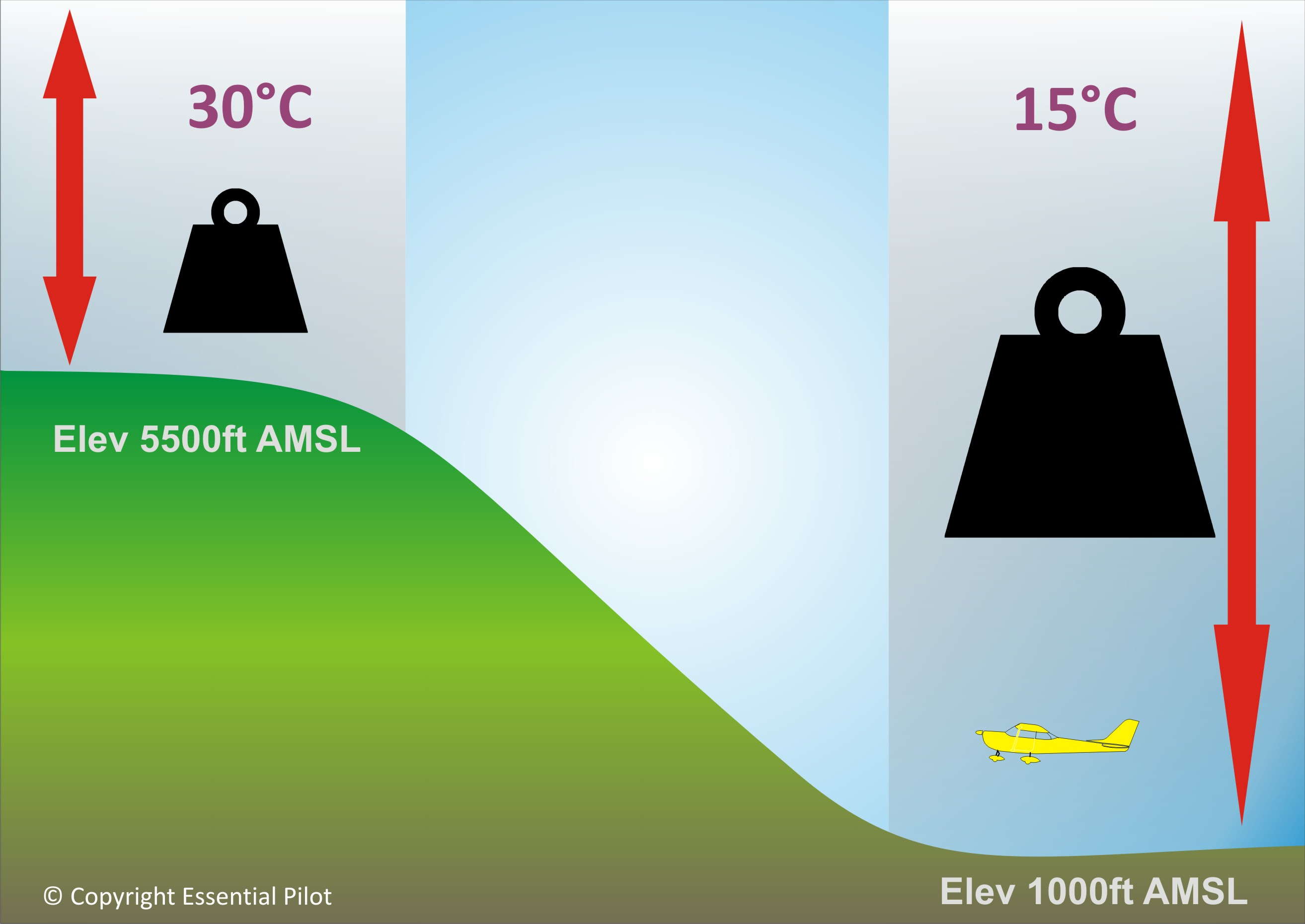Select PageThe effects of a high density altitude will seldom be realised until it is too late. This poses a particular danger to pilots operating in high altitude areas during the hot Summer months, and I am going to explain to you why, but let’s first explore what density altitude means.

Starting with pressure altitude, this is the indicated altitude on your altimeter when you enter the local QNH or the standard 1013 on the subscale. It is the altitude of aerodrome or place where you are at, adjusted for local pressure which is continuously changing along with the high and low pressure systems which pass over an area. As the column of air over a low lying area is much greater than that of a higher lying area, it will exert  greater pressure, which means that (according to the lift formula which we all struggle to remember!), your aircraft will produce more lift in an area of high pressure, for example at a coastal town. One may get excited at the prospect of your C152  performing like a jet down at the coast, which, although a bit exaggerated, is somewhat true. However, pressure is not the only external factor affecting aircraft performance.

Temperature, as well as humidity, to a lesser degree, is the next significant factor which affects aircraft performance. As temperature rises, air molecules rise and expand away from each other (think of a hot air balloon), resulting in the air becoming less dense – another essential factor in the production of lift. Simply put, consider the resistance offered by the standard soggy bath sponge(low density) and a piece of high-density foam. Aircraft optimally require higher density to generate better lift – hot, humid air is a lot like the soggy bath sponge with big holes, it offers little in terms of ‘springyness’. In comes density altiude! Density altitude is pressure altitude corrected for non-standard temperature according to ISA (International Standard Atmosphere), which means that any temperature which is not 15 degrees celcius, will produce an altitude reading which does not correspond to the ISA equivalent.

So how do we calculate density altitude in order to know how our aircraft will perform? There are a number of ways, but this is one I created using the mnemonic ‘PLASMA/S‘:
Firstly, let’s use an example of an airfield that has an elevation of 5500ft, with a temperature of 30 degrees celcius, and a a QNH of 1027

P –  Pressure Altitude – Calculate this by taking the QNH and the standard pressure at sea level of 1013, and subtract accordingly. E.g. 1027 – 1013 = 14 hPa x 30ft = 420ft (the difference). IF the actual QNH is HIGHER than 1013, then you need to SUBTRACT the difference from the airfield elevation: 5500ft – 420ft = 5080ft. Do the opposite if the QNH is lower i.e. add the difference.

LLapse Rate – Now, as you know, for every 1000ft we climb into the atmosphere, the temperature generally decreases by 2 degrees celcius (1.98), so now we need to apply this temperature decrease to our pressure altitude of 5080ft (5000ft). Take 5000 x -2 deg per 1000ft , equals -10 deg C

AAdd – Add this temperature to the ISA standard of +15 deg C: -10 + 15 = +5 deg

S –  Subtract – Subtract this from the OAT (outside air temperature): +30 deg – +5 deg = +25 deg. If the temperature deviation happens to be higher than the OAT, then you will want to ADD the two.

MMultiply – Multiply by 120 in order to get feet: 25 x 120 = 3000ft. This is the pressure difference.

AAdd – Add the difference to the pressure altitude you worked out in the beginning, if the current temperature is HIGHER than the standard of 1013. 5000ft + 3000ft = 8000ft. If the current temperature is LOWER, then subract. The final sum is the density altitude!

You can now see what an effect density altitude will have on your aircraft – if your aircraft is set for a pressure altitude of 5000ft, it will actually perform as though it were at 8000ft!

If that seems all too much for you to remember, then try this quick formula:

Quick Calculation

DA = PA + (C x 120)
DA = Density Altitude
PA = Pressure Altitude
C = °C above ISA (Deviation)

For example:
PA = 2000ft
OAT Temperature = 25°C

Here is how to do it:
The ISA temperature at 2000ft is 11°C therefore the difference (deviation) between the ISA and the actual OAT is 14°C
DA = 2000 + (14 x 120)
= 3680ft

So, how will density altitude affect your aircraft’s performance? Firstly, you need to check on your aircraft’s performance graphs and check the result as though your aircraft is actually at 8000ft – can it actually take off at this altitude? If your aircraft limit happens to be 8000ft, then it becomes obvious that it will simply not take off! If the density altitude is below the limit, but still on the high side, be prepared that it will take a longer time to lift off of the ground. Imagine a fully loaded plane with full fuel and passengers and you have a recipe for disaster!

Be safe out there 🙂